# Meaning Of Integration

Go back to  'Integration Basics'

Instead of starting right away with a list of (scary-looking!) integration formulae, we will first try to understand the physical significance of integration and why it is required at all; this will then lead to a realisation of how powerful integration really is and how indispensable it is as a tool in not only mathematics but almost all other sciences.

Mathematicians, before calculus was discovered, were interested in two issues related to an arbitrary curve.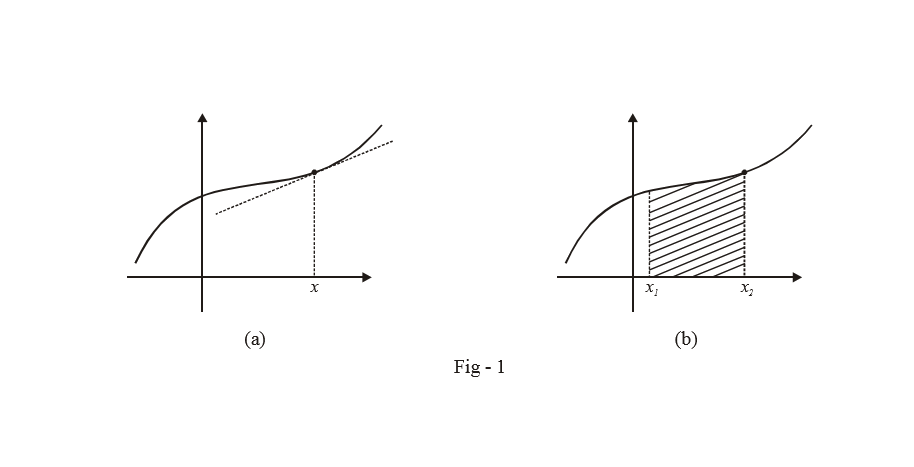How do we find the slope of the tangent to the curve at an arbitrary x? And, how do we find the area under the curve between any two arbitrary values of $$x,{x_1}$$ and $${x_2}.$$

The first question lead to Differential Calculus which we have already studied in quite detail in the previous units. The second question, of the area under the curve, gave rise to Integral Calculus, which is the subject of this and some subsequent chapters.

What do you think would be a good way of evaluating the area under an arbitrary curve $$y = f\left( x \right)$$  from  $$x = a$$ to $$x = b$$?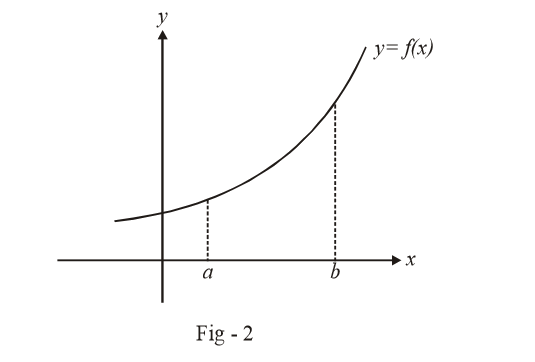One intuitive way would be to divide this area into a finite number of rectangles which approximate this required area, as shown below: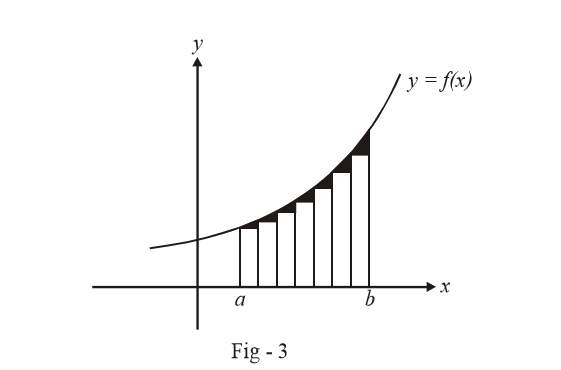For example, we have approximated the required area with 7 rectangles above; the sum of the areas of these 7 rectangles (the area of rectangle is simply width × height) then gives us an approximate value of the area under $$y = f\left( x \right).$$

How can we make this approximation more accurate?

We have taken only 7 rectangles between $$x = a$$ and$$x = b$$. Suppose we had taken a 1000 rectangles. It is intuitively clear that the sum of the areas these 1000 rectangles would much better approximate the actual area under the curve, than only 7 rectangles. In other words, the “left-out area” (the shaded area in Fig. 3, which represents the difference between area under the curve and the sum of areas of all the rectangles) would be much smaller in case of a 1000 rectangles, than only 7 rectangles.

The key to a better approximation lies, therefore, in increasing n, the number of rectangles between $$x = a$$ and  $$x = b.$$

Let us now write down $${A_n}$$, the sum of the area of the n rectangles between $$x = a$$ and $$x = b$$ for$$y = f\left( x \right)$$ .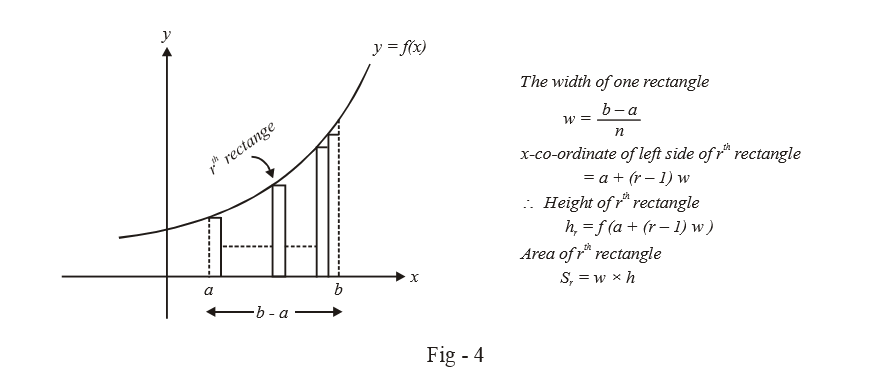From the geometry of the figure above and the explanation provided alongside:

\begin{align}&{A_n} = \sum\limits_{r = 1}^n {{S_r}} \\\,\,\,\,\,\, &\quad\;= \sum\limits_{r = 1}^n {w.f\left( {a + \left( {r - 1} \right)w} \right)} \\\,\,\,\,\, &\quad\;= \left( {\frac{{b - a}}{n}} \right)\sum\limits_{r = 1}^n {\,\,\,\,\,f\left\{ {a + \left( {\frac{{r - 1}}{n}} \right)\left( {b - a} \right)} \right\}} \end{align}

(Try to calculate this sum for a few simple functions like$$f\left( x \right) = x$$; $$f\left( x \right) = {x^2}$$ etc.)

Let the actual area under the curve be A. We have discussed that the approximation by sum will become more accurate as the number of rectangles becomes larger. As should become closer and closer to A.

$A = \mathop {\lim }\limits_{n \to \infty } {A_n} = \mathop {\lim }\limits_{n \to \infty } \left\{ {\sum\limits_{r = 1}^n {{S_r}} } \right\}$

This process is what is known as integration. We ‘integrated’ the areas of all the individual rectangles to get the area under the curve. The notation that is used for this process is: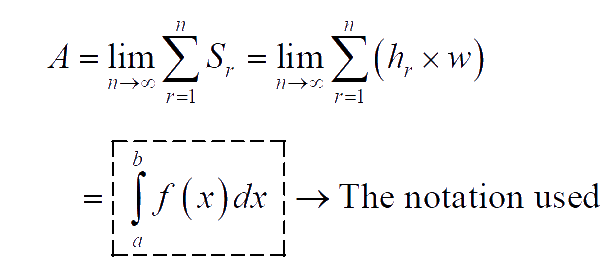Observe carefully the notation and see how each symbol corresponds to the expression just above it: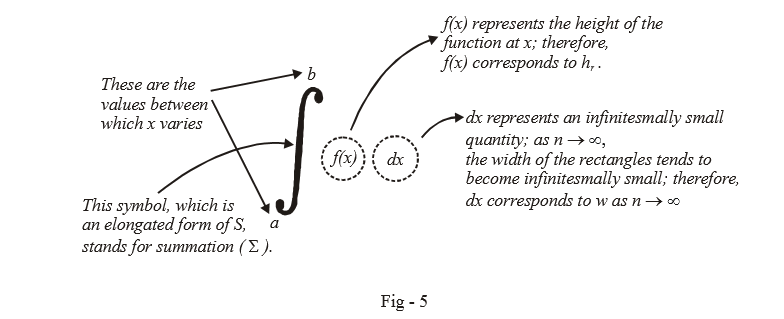It should be clear now that integration is nothing more than summation (in the limit $$n \to \infty$$).

Let us apply all this to a concrete example now.  Suppose that we want to find the area under the curve $$f\left( x \right) = {x^2}$$ between $$x = 0$$ and $$x = 3$$.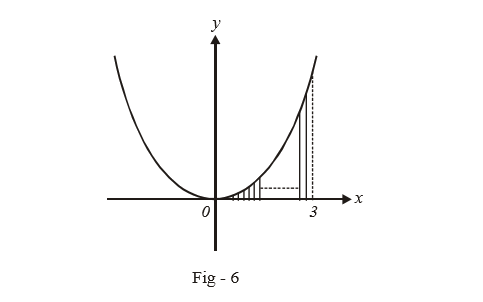We divide the interval [0, 3], into n rectangles as shown. The width of each rectangle is

$w = \frac{3}{n}$

The height of the $${r^{th}}$$ rectangle is

\begin{align}&{h_r} = f\left( {0 + \left( {r - 1} \right) \cdot \frac{3}{n}} \right)\\\,\,\,\,\, &\quad= f = \frac{9}{{{n^2}}}{\left( {r - 1} \right)^2}\\\,\,\,\,\, &\quad= \left\{ {\frac{3}{n}\left( {r - 1} \right)} \right\}\\ \end{align}

Therefore, the sum of the areas of the n rectangles is:

\begin{align}&{A_n} = \sum\limits_{r = 1}^n {w \times {h_r}} \\\,\,\,\,\,\, &\quad\;= \sum\limits_{r = 1}^n {\frac{{27}}{{{n^3}}}{{\left( {r - 1} \right)}^2}} \\\,\,\,\,\,\, &\quad\;= \frac{{27}}{{{n^3}}}\sum\limits_{r = 1}^n {{{\left( {r - 1} \right)}^2}} \\\,\,\,\,\, &\quad\;= \frac{{27}}{{{n^3}}}\frac{{\left( {n - 1} \right)\left( n \right)\left( {2n - 1} \right)}}{6}\\\,\,\,\,\, &\quad\;= \frac{9}{2} \cdot \left( {\frac{{2{n^3} - 3{n^2} + n}}{{{n^3}}}} \right)\\\,\,\,\, &\quad\;= \frac{9}{2}\left( {2 - \frac{3}{n} + \frac{1}{{{n^2}}}} \right)\end{align}

The precise area under the curve is, therefore:

\begin{align}&A = \mathop {\lim }\limits_{n \to \infty } {A_n}\\\,\,\,\,\, &\;\;\;= \frac{9}{2} \times 2\\\,\,\,\,\, &\;\;\;= 9.\end{align}

This result can be represented using the integration notation as

$\int\limits_0^3 {{x^2}dx = 9}$

The process by which we carried out this integration is know as integration by first principles. However, even for a simple function like $$f\left( x \right) = {x^2},$$   this process is lengthy, leave alone functions with complex expressions. Therefore, we need to develop a set of tools and formulae and a unified approach by which we can carry out integration in a faster manner.

Learn from the best math teachers and top your exams

• Live one on one classroom and doubt clearing
• Practice worksheets in and after class for conceptual clarity
• Personalized curriculum to keep up with school